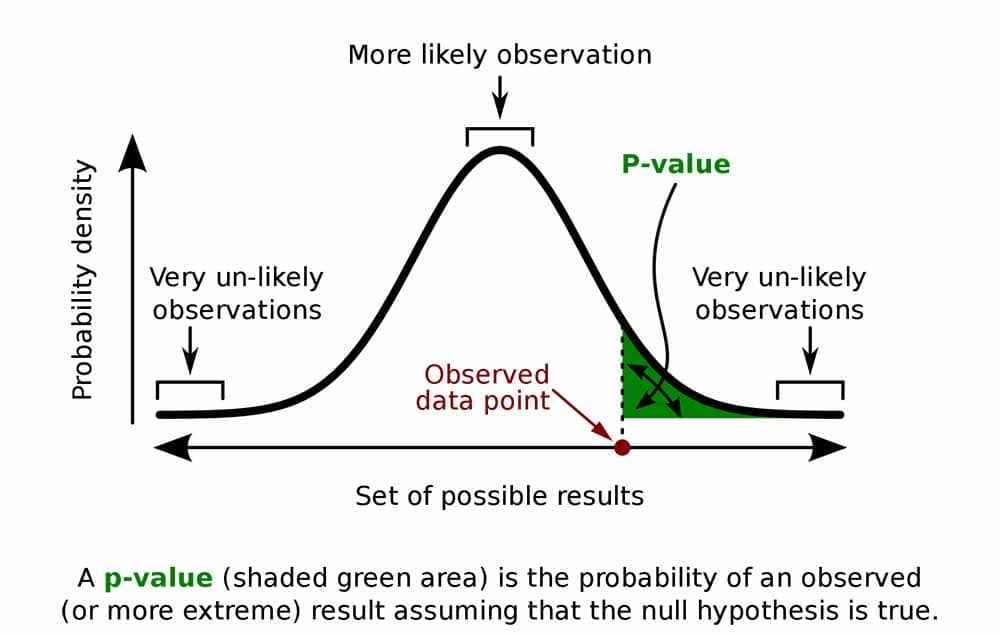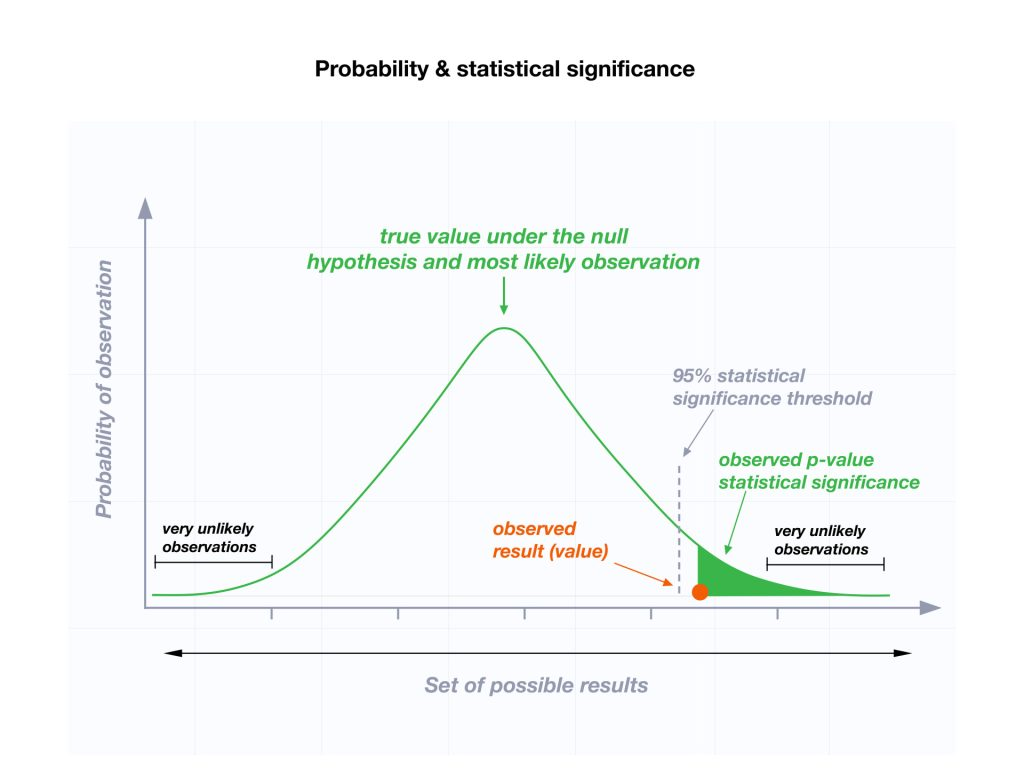#When you perform a statistical test a p -value helps you determine the significance of your results in relation to the null hypothesis.

## What a p-value tells you

The null hypothesis (H0) states that there is no relationship between the two variables being studied (one variable does not affect the other). It states the results are due to chance and are not significant in terms of supporting the idea being investigated. Thus, the null hypothesis assumes that whatever you try to prove did not happen.

The alternative hypothesis (Ha or H1) is the one you would believe if the null hypothesis is concluded to be untrue.

The alternative hypothesis states that the independent variable did affect the dependent variable, and the results are significant in terms of supporting the theory being investigated (i.e., not due to chance).

## When is it statistically significant?

A p-value, or probability value, is a number describing how likely it is that your data would have occurred by random chance (i.e. that the null hypothesis is true).

The level of statistical significance is often expressed as a p -value between 0 and 1. The smaller the p-value, the stronger the evidence that you should reject the null hypothesis.

• A p -value less than 0.05 (typically ≤ 0.05) is statistically significant. It indicates strong evidence against the null hypothesis, as there is less than a 5% probability the null is correct (and the results are random). Therefore, we reject the null hypothesis and accept the alternative hypothesis.
• However, if the p -value is below your threshold of significance (typically p < 0.05), you can reject the null hypothesis, but this does not mean that there is a 95% probability that the alternative hypothesis is true.
• The p -value is conditional upon the null hypothesis being true but is unrelated to the truth or falsity of the alternative hypothesis.
• A p -value higher than 0.05 (> 0.05) is not statistically significant and indicates strong evidence for the null hypothesis. This means we retain the null hypothesis and reject the alternative hypothesis. You should note that you cannot accept the null hypothesis; we can only reject it or fail to reject it.
• A statistically significant result cannot prove that a research hypothesis is correct (which implies 100% certainty).Instead, we may state our results “provide support for” or “give evidence for” our research hypothesis (as there is still a slight probability that the results occurred by chance and the null hypothesis was correct – e.g., less than 5%).The observed value is statistically significant (p ≤ 0.05), so the null hypothesis (N0) is rejected, and the alternative hypothesis (Ha) is accepted.

## How to report

The 6th edition of the APA style manual (American Psychological Association, 2010) states the following on the topic of reporting p-values:

“When reporting p values, report exact p values (e.g., p = .031) to two or three decimal places. However, report p values less than .001 as p < .001. The tradition of reporting p values in the form p < .10, p < .05, p < .01, and so forth, was appropriate in a time when only limited tables of critical values were available.” (p. 114)

###### Note:
• Do not use 0 before the decimal point for the statistical value p as it cannot equal 1. In other words, write p = .001 instead of p = 0.001.
• Please pay attention to issues of italics ( p is always italicized) and spacing (either side of the = sign).
• p = .000 (as outputted by some statistical packages such as SPSS) is impossible and should be written as p < .001.
• The opposite of significant is “nonsignificant,” not “insignificant.”

## Why is the p-value not enough?

A lower p -value is sometimes interpreted as meaning there is a stronger relationship between two variables. However, statistical significance means that it is unlikely that the null hypothesis is true (less than 5%).

To understand the strength of the difference between the two groups (control vs. experimental) a researcher needs to calculate the effect size.Olivia Guy-Evans

BSc (Hons), Psychology, MSc, Psychology of Education

Associate Editor for Simply Psychology

Olivia Guy-Evans is a writer and associate editor for Simply Psychology. She has previously worked in healthcare and educational sectors.Saul Mcleod, PhD

Educator, Researcher

BSc (Hons) Psychology, MRes, PhD, University of Manchester

Saul Mcleod, Ph.D., is a qualified psychology teacher with over 18 years experience of working in further and higher education.